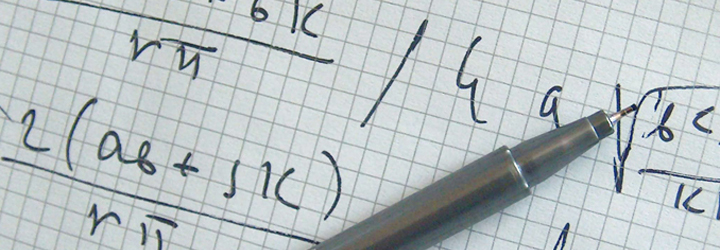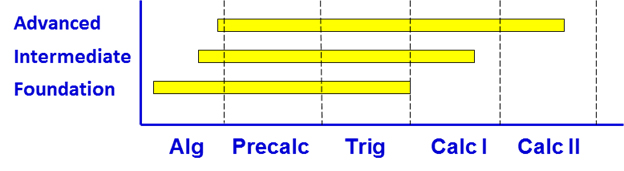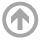Mathematics Courses

Precalculus Algebra and Trigonometry review, followed by Calc I and Intro to Calc II.

Intermediate

Precalculus Algebra and Trigonometry followed by Calc I.

Foundation

Review of basic Algebra followed by Precalculus Algebra and Trigonometry.